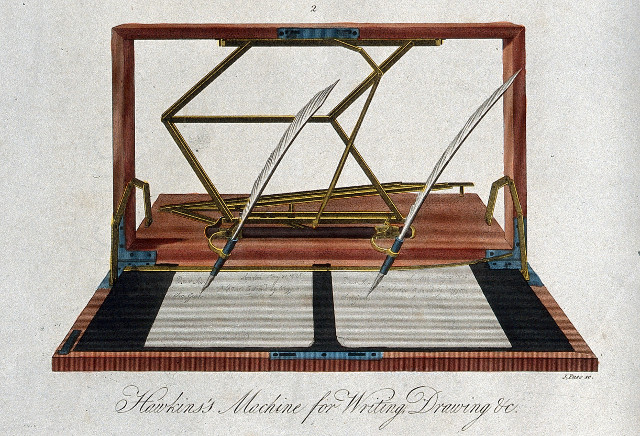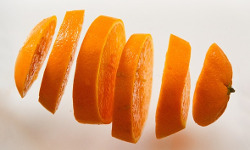# How to use the copy function

yourbasic.org/golangThe built-in copy function copies elements into a destination slice `dst` from a source slice `src`.

``func copy(dst, src []Type) int``

It returns the number of elements copied, which will be the minimum of `len(dst)` and `len(src)`. The result does not depend on whether the arguments overlap.

As a special case, it’s legal to copy bytes from a string to a slice of bytes.

``copy(dst []byte, src string) int``

## Examples

### Copy from one slice to another

``````var s = make([]int, 3)
n := copy(s, []int{0, 1, 2, 3}) // n == 3, s == []int{0, 1, 2}``````

### Copy from a slice to itself

``````s := []int{0, 1, 2}
n := copy(s, s[1:]) // n == 2, s == []int{1, 2, 2}``````

### Copy from a string to a byte slice (special case)

``````var b = make([]byte, 5)
copy(b, "Hello, world!") // b == []byte("Hello")``````

### Further readingSlices and arrays in 6 easy steps